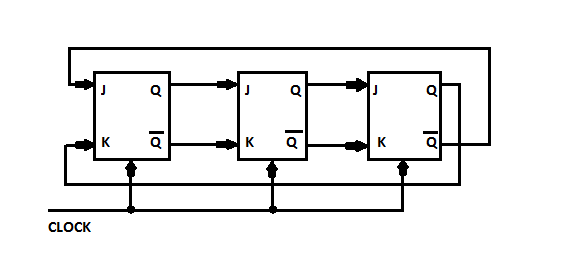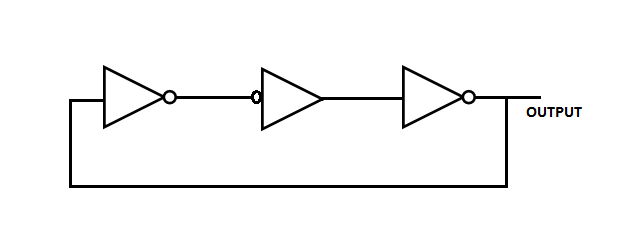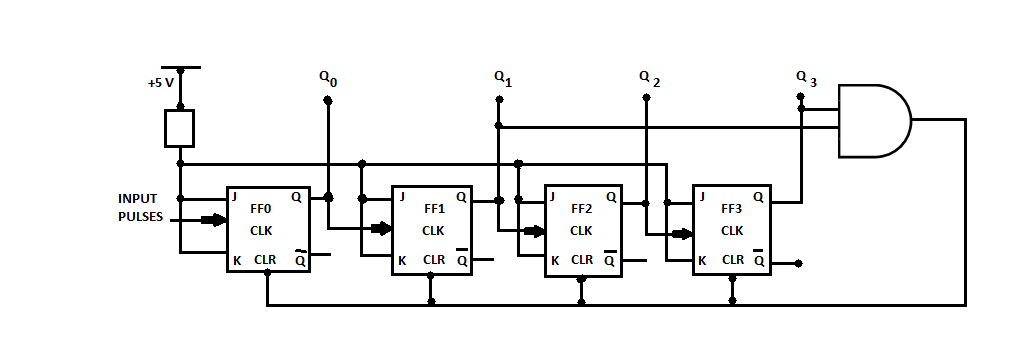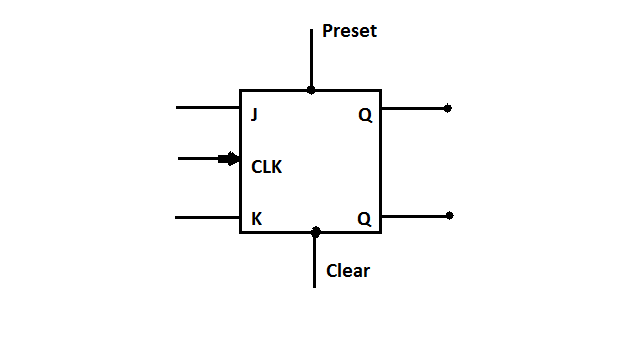# Digital Logic - Sequential Circuits

56:

For the initial state of 000, the function performed bt the arrangement of the J-K flip-flop in the given figure isA. shift register B. mod-3 counter C. mod-6 counter D. mod-2 counter Answer Report Discuss Option: C Explanation : Click on Discuss to view users comments. Write your comments here:
57:

The circuit shown in the figure given belowA. is an oscillating circuit and its output is a square wave B. is one whose output remains stable in ' 1 ' state C. is one having output remains stable ' 0 ' state D. having a single pulse of 3 times propagation delay Answer Report Discuss Option: A Explanation : Click on Discuss to view users comments. Write your comments here:
58:

In the circuit shown below  the input data is fixed at a LOW level and the output values are as shown in the figure. The number of lock pulses required to give an output of Φ Φ Φ Φ isA. 2 B. 3 C. 4 D. 5 Answer Report Discuss Option: A Explanation : Click on Discuss to view users comments. Write your comments here:
59:

In the figure given below  if Initially all flip-flops are cleared then how many clock pulses have to be applied to the system before the output from FF3 becomes a HIGH level?A. 2 B. 4 C. 6 D. 8 Answer Report Discuss Option: B Explanation : Click on Discuss to view users comments. Write your comments here:
60:

The inputs of the J-K flip-flop,  are  PRESET = CLEAR = 1 : J = K = 0
If  a single closk pulse is applied, then device willA. toggle B. set C. reset D. not change states Answer Report Discuss Option: D Explanation : Click on Discuss to view users comments. Write your comments here: